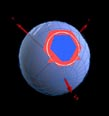HomeSDSS MirrorsDocumentationHTMCfunBASESkyServerCasJobsHelpTeamSkyServer.org - HTM

#Introduction

 Prev Next Contents

There are several domains in science where we face the problem of cataloguing and scanning through objects by object location in 3-dimensional space. In Astronomy and Earth Sciences, the coordinate system is usually spherical, so the object's coordinate is given by its localization on the surface of the unit sphere and its distance from the origin. When catalogs of objects are created, user queries mostly involve queries on these coordinates, defining areas on the unit sphere, often using complicated spherical trigonometrics.

There is a great interest to define a universal, computer-friendly index on the sphere, especially in Astronomy, where the ancient 'index' of stellar constellations is still in common use. The index we present here conveniently maps regions of the sphere to unique identifying names, that can be used by itself as a reference. The mapping uses only elementary spherical geometry to identify a certain area. This provides universality, which is essential for cross-referencing across different datasets.

We call the scheme presented here Hierarchical Triangular Mesh (HTM). This technique to subdivide the sphere into spherical triangles presented here is a recursive process. At each level of recursion the area of the triangles is roughly the same, which is a major advantage over the usual spherical coordinate system subdivisions where we have to deal with different cell sizes and singularities around the poles. Also, in areas with high data density, the recursion process can be applied to a higher level than in areas where data points are rare. This enables us to structure uneven data distributions into equal-sized bins.Figure 1: Subdividing the sphere, all triangles planar for simplicity.
Triangle sides are always great circle segments.

This scheme for subdividing the sphere has been advocated earlier . Some methodologies can be found in  The idea of the HTM has been described in , and in more detail in .

 Prev Next Contents

William O'Mullane
Last Modified :Tuesday, December 26, 2006 at 3:52:09 PM , \$Revision 1.4 \$